# Rhombus

Here we will learn about a rhombus, including what it is and how to identify it.

Students will first learn about a rhombus as part of geometry in 4 th grade.

## What is a rhombus?

A rhombus is a geometric shape with the following properties:

• Four congruent sides
• Four vertices
• Two pairs of opposite parallel sides
• Two pairs of congruent opposite angles

For example,

Let’s look at each of the properties of a rhombus.

• Four congruent sides:

Since a rhombus is a type of quadrilateral, it has four sides. All the sides are equal lengths (congruent). This can be shown with a tick mark on each side or with given measurements. Since all the sides of a rhombus are equal, it is an equilateral quadrilateral.

• Four vertices:

The sides of a rhombus come together at points called vertices. Since there are four sides, there are four vertices.

• Two pairs of opposite parallel sides:

Since a rhombus is a type of parallelogram, the opposite sides will be the same distance apart and never intersect.

• Two pairs of congruent opposite angles:

Since the pairs of opposite sides are parallel, the opposite angles formed are congruent. The curved lines within the angles are used to show equivalence.

Since a four sided polygon will always have four vertices, these properties can be combined under the property of four equal sides when classifying.

Since the two pairs of opposite parallel sides create two congruent opposite angles, these properties can be combined under the property of two pairs of opposite parallel sides when classifying.

Finally, a square is a special type of rhombus. The square shape fits all the properties of a rhombus.

Note on vocabulary: More than one rhombus can be called ‘rhombuses’ or ‘rhombi.’

### What is a rhombus?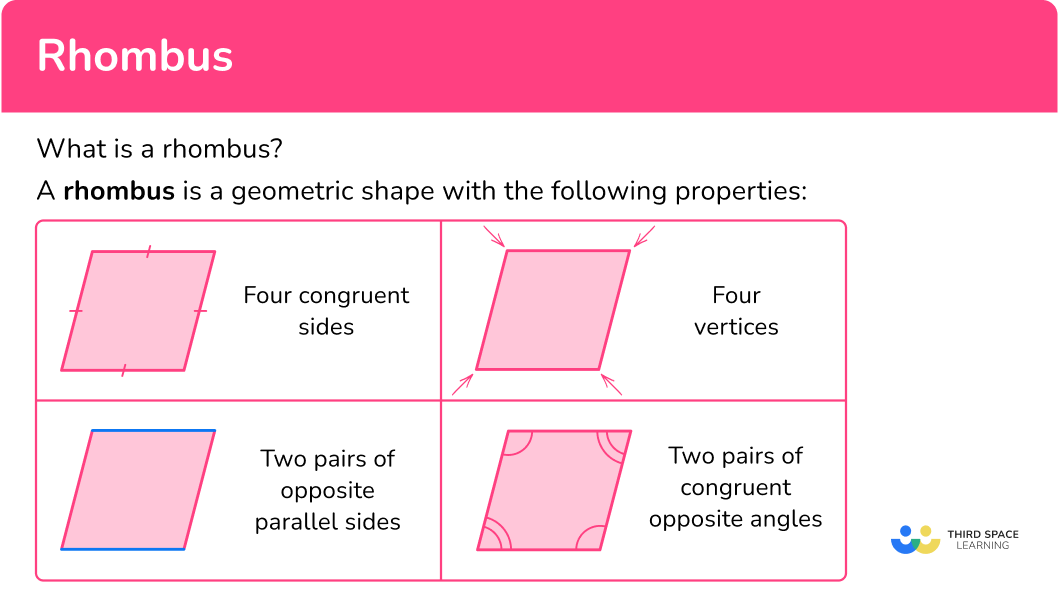## Common Core State Standards

How does this relate to 3 rd grade math to 6 th grade math?

• Grade 3 – Measurement and Data (3.MD.8)
Solve real world and mathematical problems involving perimeters of polygons, including finding the perimeter given the side lengths, finding an unknown side length, and exhibiting rectangles with the same perimeter and different areas, or with the same area and different perimeters.

• Grade 4 – Geometry (4.G.A.2)
Classify two-dimensional figures based on the presence or absence of parallel or perpendicular lines, or the presence or absence of angles of a specified size. Recognize right triangles as a category, and identify right triangles.

• Grade 5 – Geometry (5.G.B.3)
Understand that attributes belonging to a category of two-dimensional figures also belong to all subcategories of that category. For example, all rectangles have four right angles and squares are rectangles, so all squares have four right angles.

• Grade 5 – Geometry (5.G.B.4)
Classify two-dimensional figures in a hierarchy based on properties.

• Grade 6 – Geometry (6.G.1)
Find the area of right triangles, other triangles, special quadrilaterals, and polygons by composing into rectangles or decomposing into triangles and other shapes; apply these techniques in the context of solving real-world and mathematical problems.

## How to identify a rhombus

In order to identify a rhombus:

1. Look for a shape with four equal sides.
2. Decide if the shape has two pairs of opposite parallel sides.

## Rhombus examples

### Example 1: identifying a shape

Is the shape a rhombus?

1. Look for a shape with four equal sides.

The shape has four equal sides.

2Decide if the shape has two pairs of opposite parallel sides.

The opposite sides are the same distance apart and will never intersect.

The shape is a rhombus.

### Example 2: identifying a shape

Is the shape a rhombus?

The shape has four equal sides.

The opposite sides are the same distance apart and will never intersect.

The shape is a rhombus. It is also classified as a square.

### Example 3: identifying a shape

Is the shape a rhombus?

The shape does NOT have four equal sides.

The shape is NOT a rhombus.

### Example 4: identifying a shape

The sides of a polygon are 7 \, cm, 7 \, cm, 7 \, cm, and 7 \, cm , and the opposite sides are parallel. Is the shape a rhombus?

The shape has four equal sides.

Yes, the statement says “opposite sides are parallel.”

The shape is a rhombus.

Note: We don’t know anything about the angles, so the overall form of the rhombus is not clear. It could be…

Or any other angle measurements between 0^{\circ} and 180^{\circ}.

### Example 5: finding rhombuses in geometric shapes

How many rhombuses are formed by the geometric shape?

The shapes highlighted have four equal sides.

The opposite sides are the same distance apart and will never intersect.

There are 4 rhombuses in the geometric shape.

### Example 6: finding rhombuses in the real world

How many rhombuses are in the image below?

The shape of the road sign highlighted has four equal sides.

The opposite sides are the same distance apart and will never intersect.

There is one rhombus in the image.

### How to calculate the perimeter and area of a rhombus

In order to calculate the perimeter and area of a rhombus:

1. Identify the side lengths and the height.
2. Calculate.

### Example 7: calculating perimeter of a rhombus

A rhombus has a side length of 6.7 \, cm. What is the perimeter?

A rhombus has 4 equal sides, so all sides are 6.7 \, cm. The height is not needed to calculate perimeter.

Perimeter is the distance around the outside of the rhombus, so add each side.

6.7+6.7+6.7+6.7=26.8 \, cm

### Example 8: calculating area of a rhombus

What is the area of the rhombus?

The sides of the rhombus are 10.2 \, cm and the height is 8.1 \, cm.

Area is the space within a rhombus, which is found by multiplying the base and height.

10.2 \times 8.1 = 82.62 \, cm^2

### Teaching tips for rhombus

• Printable worksheets that include real world drawings or images are a good way for students to practice identifying rhombuses. They can also use a tool to measure each side, to create justifications for whether or not a shape is a rhombus.

• There are many real life items that are in the shape of a rhombus. Encourage students to look for examples of rhombuses everywhere they go.

### Easy mistakes to make

• Mistaking a rectangle for a rhombus
A rectangle has parallel opposite sides, but all the sides are not equal length, so it is NOT a rhombus.

• Forgetting the interior angles can be any measure between \bf{0}^{\circ} and \bf{180}^{\circ}
Some commonly shown rhombuses are…
Angles of 45^{\circ} and 135^{\circ} and Angles of 90^{\circ}

However, the interior angles of a rhombus can vary from >0^{\circ} to <180^{\circ} .
For example,
From angles close to 0^{\circ} and 180^{\circ} to angles close to 90^{\circ}.

• Thinking adjacent angles in a rhombus are always equal
Adjacent sides are equal, but not necessarily the angles. The only time the adjacent angles are equal is when the rhombus can also be classified as a square.
For example,

### Practice rhombus questions

1. Which geometric shape is a rhombus?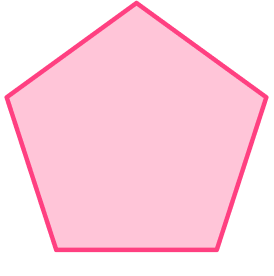Thinking about the properties, a rhombus has 4 sides, so it can’t be this shape:The side lengths of a rhombus are equal, so it cannot be these two shapes:The opposite sides of a rhombus are parallel:This shape is a rhombus:2. Which geometric shape is a rhombus?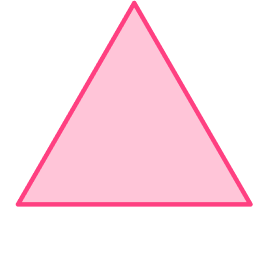Thinking about the properties, a rhombus has sides of equal length, so it can’t be these two shapes:The opposite sides of a rhombus are parallel, so it can’t be this shape: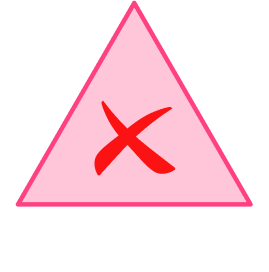Note: This shape also only has 3 sides and a rhombus has 4.

This shape is a rhombus:3. Is this shape a rhombus? Why or why not?No, it does not have 4 sides and 4 right angles.Yes, it has 4 equal sides and pairs of parallel sides.No, it does not have 4 equal sides and pairs of parallel sides.Yes, it has 4 sides and 4 right angles.A rhombus must have 4 equal sides…and pairs of parallel sides.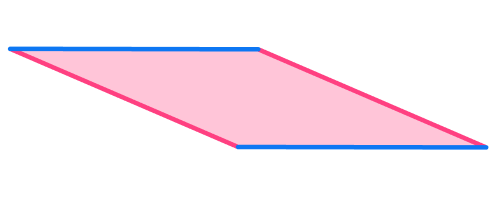This shape is a rhombus.

4. The sides of a polygon are: 4 \, m, 4 \, m, 4 \, m, 4 \, m, 4 \, m, and 4 \, m , and the opposite sides are parallel. Is the shape a rhombus? Why?

Yes, it has all equal sides.No, it does not have all equal sides.Yes, it has exactly four sides.No, it does not have exactly four sides.The shape has six equal sides. The shape is a hexagon, not a rhombus.

5. How many rhombuses are formed by the geometric shape?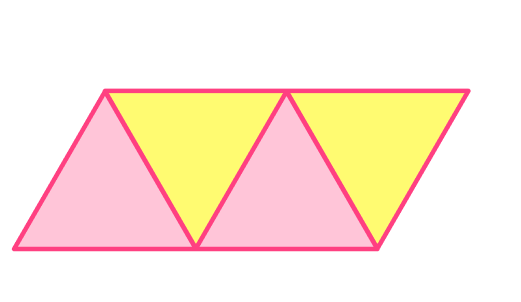4321The shapes highlighted have four equal sides and the opposite sides are parallel.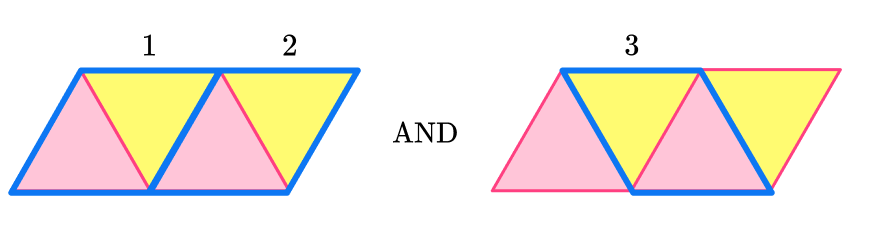There are 3 rhombuses in the geometric shape.

6. How many rhombuses are in the flag of the state of Arkansas?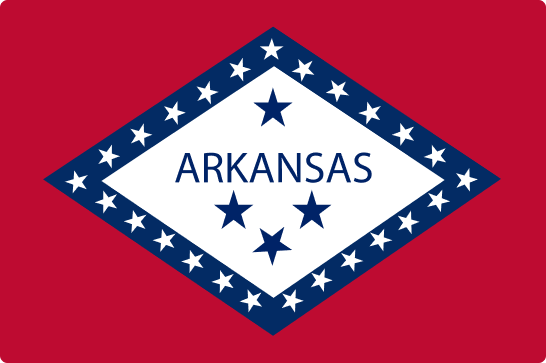0123The shapes highlighted have four equal sides and the opposite sides are parallel.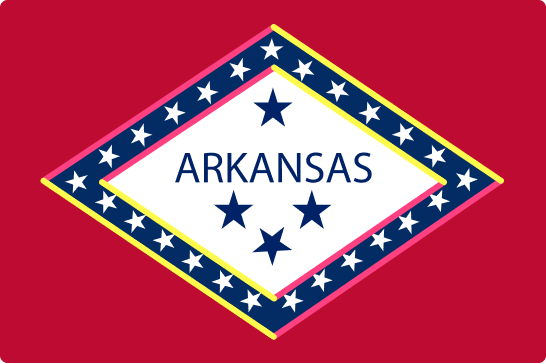There are 2 rhombuses in the flag.

7. What is the perimeter of the rhombus?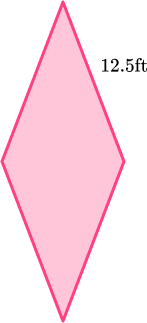12.5 ft25 ft50 ft156.25 ftA rhombus has 4 equal sides, so all sides are 12.5 ft .

Perimeter is the distance around the outside of the rhombus, so add each side.

12.5 + 12.5 + 12.5 + 12.5 = 50 ft

8. What is the area of the rhombus?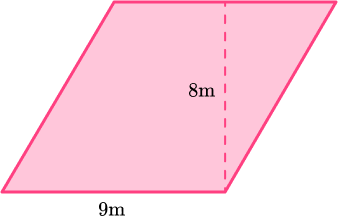17 m36 m34 m72 mThe sides of the rhombus are 9 m and the height is 8 m.

Area is the space within a rhombus, which is found by multiplying the base and height.

9 × 8 = 72 m^2

## Rhombus FAQs

How do you calculate the perimeter of a rhombus and the area of a rhombus?

The perimeter is the distance around the outside of a rhombus, so you can add all the sides or multiply one side length by 4. The perimeter is measured in units.

Perimeter = a + a + a + a OR Perimeter = 4a

The area of the rhombus is the space inside the rhombus and is calculated by multiplying the base times the height. The area is measured in square units.

Area of rhombus = b × h

What is the sum of the interior angles of a rhombus?

All four angles always add to be 360 degrees in total.

Does a rhombus always have perpendicular bisectors?

Yes, no matter the length of the diagonals, the diagonals of a rhombus bisect each other at 90 degree angles.

Does a rhombus fit the properties of a parallelogram?

Yes, a rhombus is a special case of a parallelogram. It is a parallelogram that has all congruent sides.

What is a lozenge?

A lozenge is another name for a certain type of rhombus. It has two acute angles and two obtuse angles.

What are the origins of the word rhombus?

Rhombus comes from the ancient greek word ‘rhombos.’

## Still stuck?

At Third Space Learning, we specialize in helping teachers and school leaders to provide personalized math support for more of their students through high-quality, online one-on-one math tutoring delivered by subject experts.

Each week, our tutors support thousands of students who are at risk of not meeting their grade-level expectations, and help accelerate their progress and boost their confidence.